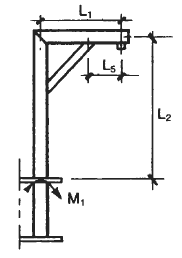### Lifting Boom, Davits Application and Design Equations and Calculator

Lifting boom equations and calculator. Davits refer to single mechanical arms with a winch for lowering and raising objects. A davit is commonly used system to used to lower an emergency life boat from a ship to the embarkation level to be boarded. The davit has falls (now made of wire, used to be made of manilla) that are used to lower the lifeboat into the water.

All calculators require a Premium Membership

 Notations Used CV = vertical impact factor, 1.5-1.8 Ch, = horizontal impact factor, 0.2-0.5 fa = axial stress, (psi, N/mm2) fb = bending stress, (psi, N/mm2) fh = horizontal force (lbs, N) fv = vertical force, (lbs, N) Fa = allowable axial stress, (psi, N/mm2) Fb = allowable bending stress, (psi, N/mm2) Fr = radial load, (lbs, N) Fve = equivalent radial load, (lbs, N) Fv = minimum specified yield stress, (psi, N/mm2) M1 = bending moment in mast at top guide or support, (in-lb, mm-N) M2 = maximum bending moment in curved davit. (in-lb, mm-N) M3 = bending moment in boom, (in-lb, mm-N) Mx = longitudinal moment, (in-lb, mm-N) Mø = circumferential moment, (in-lb, mm-N) WD = total weight of davit (lbs, N) Wl = weight of boom and brace, (lbs, N) WL = maximum rated capacity, (lbs, N) a , ß , K = stress coefficients P = axial load, (lbs, N) I = moment of inertia, (in4, mm4) A = cross-sectional area, (in2, mm2) Z = section modulus, (in3, mm3) r = least radius of gyration, (in, mm) tp = wall thickness of pipe davit, (in, mm) a = outside radius of pipe, (in, mm).Figure 1, Type of Davit Rigging

Moments and Forces Equations on DavitsBending moment in davit mast, Ml.Radial force at guide and support, Fr.Fr, is maximum when davit rotation ø is at 0°, for other rotations:Circumferential moment at guide and support, MøAxial load on davit mast, P.Longitudinal moment at support, Mx

Mx = PL4Figure 2, Davit Selection TableFigure 3 Type A Davit Equations Axial stress mast fa= P/A Bending stress mast fh = Ml/Z Combined stress mastBending stress boomType B Davit EquationsFigure 4 Axial stress mast fa= P/A Bending moment, M2Bending stressCombined stressMembership Register | LoginHomeEngineering Book StoreEngineering ForumExcel App. DownloadsOnline Books & ManualsEngineering NewsEngineering VideosEngineering CalculatorsEngineering ToolboxGD&T Training Geometric Dimensioning TolerancingDFM DFA TrainingTraining Online EngineeringAdvertising CenterCopyright Notice